# HYBRIDIZATION (1).pdf

15 Jan 2023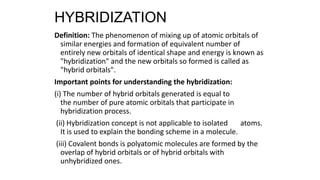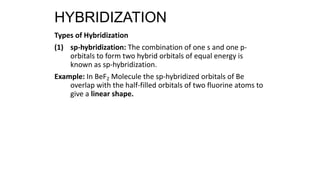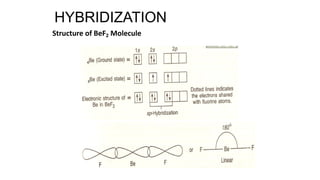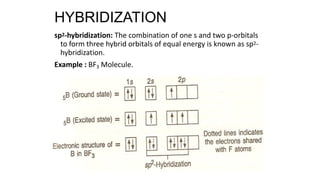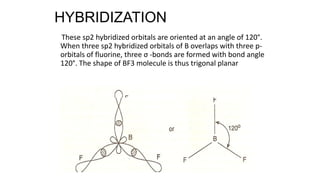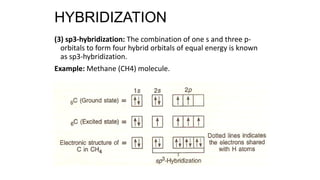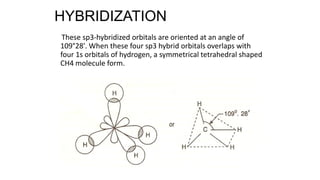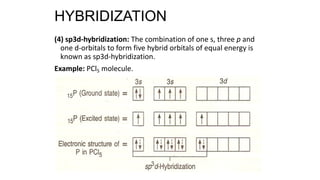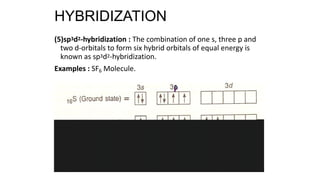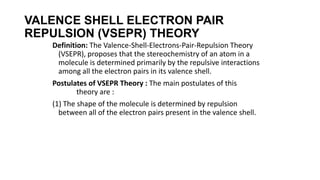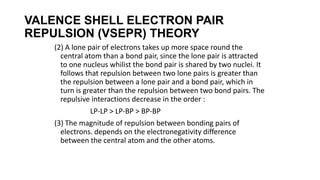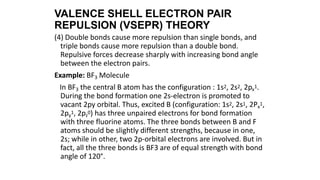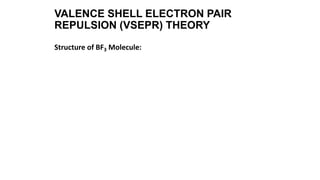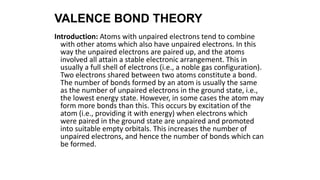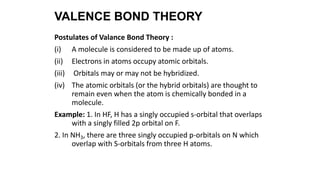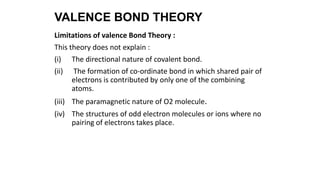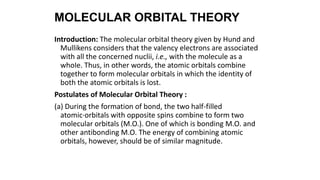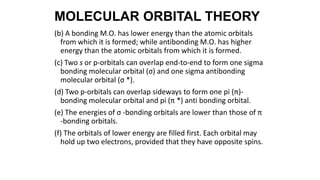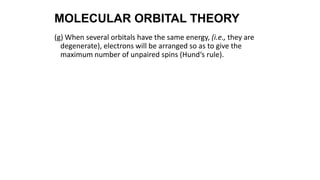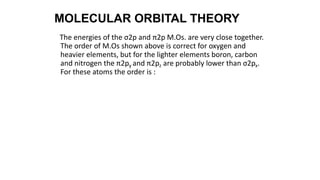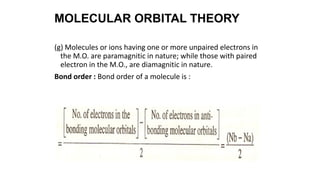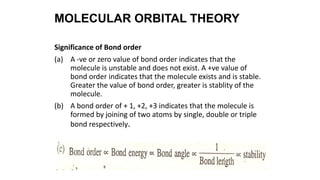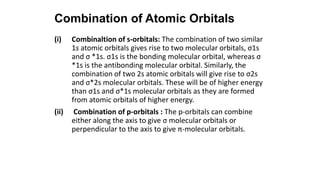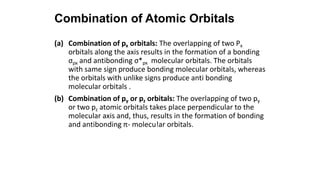1 sur 48

### HYBRIDIZATION (1).pdf

• 1. HYBRIDIZATION Definition: The phenomenon of mixing up of atomic orbitals of similar energies and formation of equivalent number of entirely new orbitals of identical shape and energy is known as "hybridization" and the new orbitals so formed is called as "hybrid orbitals". Important points for understanding the hybridization: (i) The number of hybrid orbitals generated is equal to the number of pure atomic orbitals that participate in hybridization process. (ii) Hybridization concept is not applicable to isolated atoms. It is used to explain the bonding scheme in a molecule. (iii) Covalent bonds is polyatomic molecules are formed by the overlap of hybrid orbitals or of hybrid orbitals with unhybridized ones.
• 2. Types of Hybridization (1) sp-hybridization: The combination of one s and one p- orbitals to form two hybrid orbitals of equal energy is known as sp-hybridization. Example: In BeF2 Molecule the sp-hybridized orbitals of Be overlap with the half-filled orbitals of two fluorine atoms to give a linear shape. HYBRIDIZATION
• 3. Structure of BeF2 Molecule HYBRIDIZATION
• 4. sp2-hybridization: The combination of one s and two p-orbitals to form three hybrid orbitals of equal energy is known as sp2- hybridization. Example : BF3 Molecule. HYBRIDIZATION
• 5. These sp2 hybridized orbitals are oriented at an angle of 120°. When three sp2 hybridized orbitals of B overlaps with three p- orbitals of fluorine, three σ -bonds are formed with bond angle 120°. The shape of BF3 molecule is thus trigonal planar HYBRIDIZATION
• 6. (3) sp3-hybridization: The combination of one s and three p- orbitals to form four hybrid orbitals of equal energy is known as sp3-hybridization. Example: Methane (CH4) molecule. HYBRIDIZATION
• 7. These sp3-hybridized orbitals are oriented at an angle of 109°28'. When these four sp3 hybrid orbitals overlaps with four 1s orbitals of hydrogen, a symmetrical tetrahedral shaped CH4 molecule form. HYBRIDIZATION
• 8. (4) sp3d-hybridization: The combination of one s, three p and one d-orbitals to form five hybrid orbitals of equal energy is known as sp3d-hybridization. Example: PCl5 molecule. HYBRIDIZATION
• 9. (5)sp3d2-hybridization : The combination of one s, three p and two d-orbitals to form six hybrid orbitals of equal energy is known as sp3d2-hybridization. Examples : SF6 Molecule. HYBRIDIZATION
• 10. VALENCE SHELL ELECTRON PAIR REPULSION (VSEPR) THEORY Definition: The Valence-Shell-Electrons-Pair-Repulsion Theory (VSEPR), proposes that the stereochemistry of an atom in a molecule is determined primarily by the repulsive interactions among all the electron pairs in its valence shell. Postulates of VSEPR Theory : The main postulates of this theory are : (1) The shape of the molecule is determined by repulsion between all of the electron pairs present in the valence shell.
• 11. (2) A lone pair of electrons takes up more space round the central atom than a bond pair, since the lone pair is attracted to one nucleus whilist the bond pair is shared by two nuclei. It follows that repulsion between two lone pairs is greater than the repulsion between a lone pair and a bond pair, which in turn is greater than the repulsion between two bond pairs. The repulsive interactions decrease in the order : LP-LP > LP-BP > BP-BP (3) The magnitude of repulsion between bonding pairs of electrons. depends on the electronegativity difference between the central atom and the other atoms. VALENCE SHELL ELECTRON PAIR REPULSION (VSEPR) THEORY
• 12. (4) Double bonds cause more repulsion than single bonds, and triple bonds cause more repulsion than a double bond. Repulsive forces decrease sharply with increasing bond angle between the electron pairs. Example: BF3 Molecule In BF3 the central B atom has the configuration : 1s2, 2s2, 2px 1. During the bond formation one 2s-electron is promoted to vacant 2py orbital. Thus, excited B (configuration: 1s2, 2s1, 2Px 1, 2py 1, 2pz 0) has three unpaired eIectrons for bond formation with three fluorine atoms. The three bonds between B and F atoms should be slightly different strengths, because in one, 2s; while in other, two 2p-orbital electrons are involved. But in fact, all the three bonds is BF3 are of equal strength with bond angle of 120°. VALENCE SHELL ELECTRON PAIR REPULSION (VSEPR) THEORY
• 13. Structure of BF3 Molecule: VALENCE SHELL ELECTRON PAIR REPULSION (VSEPR) THEORY
• 14. VALENCE BOND THEORY Introduction: Atoms with unpaired electrons tend to combine with other atoms which also have unpaired electrons. In this way the unpaired electrons are paired up, and the atoms involved all attain a stable electronic arrangement. This in usually a full shell of electrons (i.e., a noble gas configuration). Two electrons shared between two atoms constitute a bond. The number of bonds formed by an atom is usually the same as the number of unpaired electrons in the ground state, i.e., the lowest energy state. However, in some cases the atom may form more bonds than this. This occurs by excitation of the atom (i.e., providing it with energy) when electrons which were paired in the ground state are unpaired and promoted into suitable empty orbitals. This increases the number of unpaired electrons, and hence the number of bonds which can be formed.
• 15. Postulates of Valance Bond Theory : (i) A molecule is considered to be made up of atoms. (ii) Electrons in atoms occupy atomic orbitals. (iii) Orbitals may or may not be hybridized. (iv) The atomic orbitals (or the hybrid orbitals) are thought to remain even when the atom is chemically bonded in a molecule. Example: 1. In HF, H has a singly occupied s-orbital that overlaps with a singly filled 2p orbital on F. 2. In NH3, there are three singly occupied p-orbitals on N which overlap with S-orbitals from three H atoms. VALENCE BOND THEORY
• 16. Limitations of valence Bond Theory : This theory does not explain : (i) The directional nature of covalent bond. (ii) The formation of co-ordinate bond in which shared pair of electrons is contributed by only one of the combining atoms. (iii) The paramagnetic nature of O2 molecule. (iv) The structures of odd electron molecules or ions where no pairing of electrons takes place. VALENCE BOND THEORY
• 17. MOLECULAR ORBITAL THEORY Introduction: The molecular orbital theory given by Hund and Mullikens considers that the valency electrons are associated with all the concerned nuclii, i.e., with the molecule as a whoIe. Thus, in other words, the atomic orbitals combine together to form molecular orbitals in which the identity of both the atomic orbitals is lost. Postulates of Molecular Orbital Theory : (a) During the formation of bond, the two half-filled atomic·orbitals with opposite spins combine to form two molecular orbitals (M.O.). One of which is bonding M.O. and other antibonding M.O. The energy of combining atomic orbitals, however, should be of similar magnitude.
• 18. (b) A bonding M.O. has lower energy than the atomic orbitals from which it is formed; while antibonding M.O. has higher energy than the atomic orbitals from which it is formed. (c) Two s or p-orbitals can overlap end-to-end to form one sigma bonding molecular orbital (σ) and one sigma antibonding molecular orbital (σ *). (d) Two p-orbitals can overlap sideways to form one pi (π)- bonding molecular orbital and pi (π *) anti bonding orbital. (e) The energies of σ -bonding orbitals are lower than those of π -bonding orbitals. (f) The orbitals of lower energy are filled first. Each orbital may hold up two electrons, provided that they have opposite spins. MOLECULAR ORBITAL THEORY
• 19. (g) When several orbitals have the same energy, (i.e., they are degenerate), electrons will be arranged so as to give the maximum number of unpaired spins (Hund‘s rule). MOLECULAR ORBITAL THEORY
• 20. The energies of the σ2p and π2p M.Os. are very close together. The order of M.Os shown above is correct for oxygen and heavier elements, but for the lighter elements boron, carbon and nitrogen the π2py and π2pz are probably lower than σ2px. For these atoms the order is : MOLECULAR ORBITAL THEORY
• 21. (g) Molecules or ions having one or more unpaired electrons in the M.O. are paramagnitic in nature; while those with paired electron in the M.O., are diamagnitic in nature. Bond order : Bond order of a molecule is : MOLECULAR ORBITAL THEORY
• 22. Significance of Bond order (a) A -ve or zero value of bond order indicates that the molecule is unstable and does not exist. A +ve value of bond order indicates that the molecule exists and is stable. Greater the value of bond order, greater is stablity of the molecule. (b) A bond order of + 1, +2, +3 indicates that the molecule is formed by joining of two atoms by single, double or triple bond respectively. MOLECULAR ORBITAL THEORY
• 23. Combination of Atomic Orbitals (i) Combinaltion of s-orbitals: The combination of two similar 1s atomic orbitals gives rise to two molecular orbitals, σ1s and σ *1s. σ1s is the bonding molecular orbital, whereas σ *1s is the antibonding molecular orbital. Similarly, the combination of two 2s atomic orbitals will give rise to σ2s and σ*2s molecular orbitals. These will be of higher energy than σ1s and σ*1s molecular orbitals as they are formed from atomic orbitals of higher energy. (ii) Combination of p-orbitals : The p-orbitals can combine either along the axis to give σ molecular orbitals or perpendicular to the axis to give π-molecular orbitals.
• 24. (a) Combination of px orbitals: The overlapping of two Px orbitals along the axis results in the formation of a bonding σpx and antibonding σ*px molecular orbitals. The orbitals with same sign produce bonding molecular orbitals, whereas the orbitals with unlike signs produce anti bonding molecular orbitals . (b) Combination of py or pz orbitals: The overlapping of two py or two pz atomic orbitals takes place perpendicular to the molecular axis and, thus, results in the formation of bonding and antibonding π- molecu!ar orbitals. Combination of Atomic Orbitals
• 25. HYBRIDIZATION Definition: The phenomenon of mixing up of atomic orbitals of similar energies and formation of equivalent number of entirely new orbitals of identical shape and energy is known as "hybridization" and the new orbitals so formed is called as "hybrid orbitals". Important points for understanding the hybridization: (i) The number of hybrid orbitals generated is equal to the number of pure atomic orbitals that participate in hybridization process. (ii) Hybridization concept is not applicable to isolated atoms. It is used to explain the bonding scheme in a molecule. (iii) Covalent bonds is polyatomic molecules are formed by the overlap of hybrid orbitals or of hybrid orbitals with unhybridized ones.
• 26. Types of Hybridization (1) sp-hybridization: The combination of one s and one p- orbitals to form two hybrid orbitals of equal energy is known as sp-hybridization. Example: In BeF2 Molecule the sp-hybridized orbitals of Be overlap with the half-filled orbitals of two fluorine atoms to give a linear shape. HYBRIDIZATION
• 27. Structure of BeF2 Molecule HYBRIDIZATION
• 28. sp2-hybridization: The combination of one s and two p-orbitals to form three hybrid orbitals of equal energy is known as sp2- hybridization. Example : BF3 Molecule. HYBRIDIZATION
• 29. These sp2 hybridized orbitals are oriented at an angle of 120°. When three sp2 hybridized orbitals of B overlaps with three p- orbitals of fluorine, three σ -bonds are formed with bond angle 120°. The shape of BF3 molecule is thus trigonal planar HYBRIDIZATION
• 30. (3) sp3-hybridization: The combination of one s and three p- orbitals to form four hybrid orbitals of equal energy is known as sp3-hybridization. Example: Methane (CH4) molecule. HYBRIDIZATION
• 31. These sp3-hybridized orbitals are oriented at an angle of 109°28'. When these four sp3 hybrid orbitals overlaps with four 1s orbitals of hydrogen, a symmetrical tetrahedral shaped CH4 molecule form. HYBRIDIZATION
• 32. (4) sp3d-hybridization: The combination of one s, three p and one d-orbitals to form five hybrid orbitals of equal energy is known as sp3d-hybridization. Example: PCl5 molecule. HYBRIDIZATION
• 33. (5)sp3d2-hybridization : The combination of one s, three p and two d-orbitals to form six hybrid orbitals of equal energy is known as sp3d2-hybridization. Examples : SF6 Molecule. HYBRIDIZATION
• 34. VALENCE SHELL ELECTRON PAIR REPULSION (VSEPR) THEORY Definition: The Valence-Shell-Electrons-Pair-Repulsion Theory (VSEPR), proposes that the stereochemistry of an atom in a molecule is determined primarily by the repulsive interactions among all the electron pairs in its valence shell. Postulates of VSEPR Theory : The main postulates of this theory are : (1) The shape of the molecule is determined by repulsion between all of the electron pairs present in the valence shell.
• 35. (2) A lone pair of electrons takes up more space round the central atom than a bond pair, since the lone pair is attracted to one nucleus whilist the bond pair is shared by two nuclei. It follows that repulsion between two lone pairs is greater than the repulsion between a lone pair and a bond pair, which in turn is greater than the repulsion between two bond pairs. The repulsive interactions decrease in the order : LP-LP > LP-BP > BP-BP (3) The magnitude of repulsion between bonding pairs of electrons. depends on the electronegativity difference between the central atom and the other atoms. VALENCE SHELL ELECTRON PAIR REPULSION (VSEPR) THEORY
• 36. (4) Double bonds cause more repulsion than single bonds, and triple bonds cause more repulsion than a double bond. Repulsive forces decrease sharply with increasing bond angle between the electron pairs. Example: BF3 Molecule In BF3 the central B atom has the configuration : 1s2, 2s2, 2px 1. During the bond formation one 2s-electron is promoted to vacant 2py orbital. Thus, excited B (configuration: 1s2, 2s1, 2Px 1, 2py 1, 2pz 0) has three unpaired eIectrons for bond formation with three fluorine atoms. The three bonds between B and F atoms should be slightly different strengths, because in one, 2s; while in other, two 2p-orbital electrons are involved. But in fact, all the three bonds is BF3 are of equal strength with bond angle of 120°. VALENCE SHELL ELECTRON PAIR REPULSION (VSEPR) THEORY
• 37. Structure of BF3 Molecule: VALENCE SHELL ELECTRON PAIR REPULSION (VSEPR) THEORY
• 38. VALENCE BOND THEORY Introduction: Atoms with unpaired electrons tend to combine with other atoms which also have unpaired electrons. In this way the unpaired electrons are paired up, and the atoms involved all attain a stable electronic arrangement. This in usually a full shell of electrons (i.e., a noble gas configuration). Two electrons shared between two atoms constitute a bond. The number of bonds formed by an atom is usually the same as the number of unpaired electrons in the ground state, i.e., the lowest energy state. However, in some cases the atom may form more bonds than this. This occurs by excitation of the atom (i.e., providing it with energy) when electrons which were paired in the ground state are unpaired and promoted into suitable empty orbitals. This increases the number of unpaired electrons, and hence the number of bonds which can be formed.
• 39. Postulates of Valance Bond Theory : (i) A molecule is considered to be made up of atoms. (ii) Electrons in atoms occupy atomic orbitals. (iii) Orbitals may or may not be hybridized. (iv) The atomic orbitals (or the hybrid orbitals) are thought to remain even when the atom is chemically bonded in a molecule. Example: 1. In HF, H has a singly occupied s-orbital that overlaps with a singly filled 2p orbital on F. 2. In NH3, there are three singly occupied p-orbitals on N which overlap with S-orbitals from three H atoms. VALENCE BOND THEORY
• 40. Limitations of valence Bond Theory : This theory does not explain : (i) The directional nature of covalent bond. (ii) The formation of co-ordinate bond in which shared pair of electrons is contributed by only one of the combining atoms. (iii) The paramagnetic nature of O2 molecule. (iv) The structures of odd electron molecules or ions where no pairing of electrons takes place. VALENCE BOND THEORY
• 41. MOLECULAR ORBITAL THEORY Introduction: The molecular orbital theory given by Hund and Mullikens considers that the valency electrons are associated with all the concerned nuclii, i.e., with the molecule as a whoIe. Thus, in other words, the atomic orbitals combine together to form molecular orbitals in which the identity of both the atomic orbitals is lost. Postulates of Molecular Orbital Theory : (a) During the formation of bond, the two half-filled atomic·orbitals with opposite spins combine to form two molecular orbitals (M.O.). One of which is bonding M.O. and other antibonding M.O. The energy of combining atomic orbitals, however, should be of similar magnitude.
• 42. (b) A bonding M.O. has lower energy than the atomic orbitals from which it is formed; while antibonding M.O. has higher energy than the atomic orbitals from which it is formed. (c) Two s or p-orbitals can overlap end-to-end to form one sigma bonding molecular orbital (σ) and one sigma antibonding molecular orbital (σ *). (d) Two p-orbitals can overlap sideways to form one pi (π)- bonding molecular orbital and pi (π *) anti bonding orbital. (e) The energies of σ -bonding orbitals are lower than those of π -bonding orbitals. (f) The orbitals of lower energy are filled first. Each orbital may hold up two electrons, provided that they have opposite spins. MOLECULAR ORBITAL THEORY
• 43. (g) When several orbitals have the same energy, (i.e., they are degenerate), electrons will be arranged so as to give the maximum number of unpaired spins (Hund‘s rule). MOLECULAR ORBITAL THEORY
• 44. The energies of the σ2p and π2p M.Os. are very close together. The order of M.Os shown above is correct for oxygen and heavier elements, but for the lighter elements boron, carbon and nitrogen the π2py and π2pz are probably lower than σ2px. For these atoms the order is : MOLECULAR ORBITAL THEORY
• 45. (g) Molecules or ions having one or more unpaired electrons in the M.O. are paramagnitic in nature; while those with paired electron in the M.O., are diamagnitic in nature. Bond order : Bond order of a molecule is : MOLECULAR ORBITAL THEORY
• 46. Significance of Bond order (a) A -ve or zero value of bond order indicates that the molecule is unstable and does not exist. A +ve value of bond order indicates that the molecule exists and is stable. Greater the value of bond order, greater is stablity of the molecule. (b) A bond order of + 1, +2, +3 indicates that the molecule is formed by joining of two atoms by single, double or triple bond respectively. MOLECULAR ORBITAL THEORY
• 47. Combination of Atomic Orbitals (i) Combinaltion of s-orbitals: The combination of two similar 1s atomic orbitals gives rise to two molecular orbitals, σ1s and σ *1s. σ1s is the bonding molecular orbital, whereas σ *1s is the antibonding molecular orbital. Similarly, the combination of two 2s atomic orbitals will give rise to σ2s and σ*2s molecular orbitals. These will be of higher energy than σ1s and σ*1s molecular orbitals as they are formed from atomic orbitals of higher energy. (ii) Combination of p-orbitals : The p-orbitals can combine either along the axis to give σ molecular orbitals or perpendicular to the axis to give π-molecular orbitals.
• 48. (a) Combination of px orbitals: The overlapping of two Px orbitals along the axis results in the formation of a bonding σpx and antibonding σ*px molecular orbitals. The orbitals with same sign produce bonding molecular orbitals, whereas the orbitals with unlike signs produce anti bonding molecular orbitals . (b) Combination of py or pz orbitals: The overlapping of two py or two pz atomic orbitals takes place perpendicular to the molecular axis and, thus, results in the formation of bonding and antibonding π- molecu!ar orbitals. Combination of Atomic Orbitals